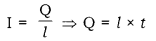# Derive the expression for the heat produced due to a current T flowing for a time interval

Derive the expression for the heat produced due to a current T flowing for a time interval ‘t’ through a resistor ‘R’ having a potential difference ‘V’ across its ends with which name is the relation known? How much heat will an instrument of 12W produce in one minute if it is connected to a battery of 12V?

Consider a resistor of resistance R. Let the current flowing through this resistor be l and the potential difference across it be V.
In time t, let Q amount of charge flows through the resistor.
Work done on moving this charge, W = VQ …(i)
According to the definition of electric current,Putting this in equation (i).
W = V × l × t